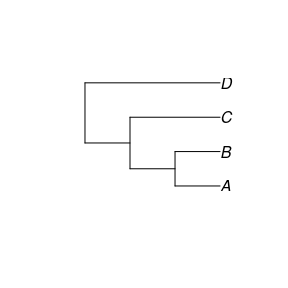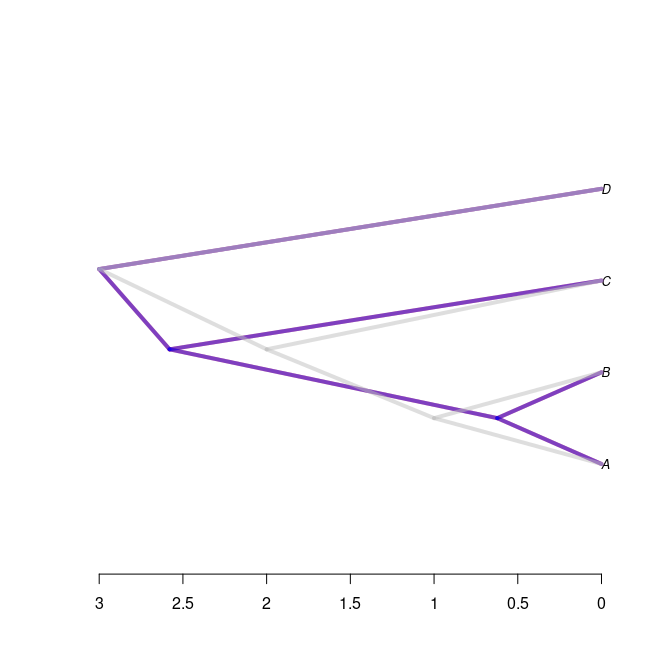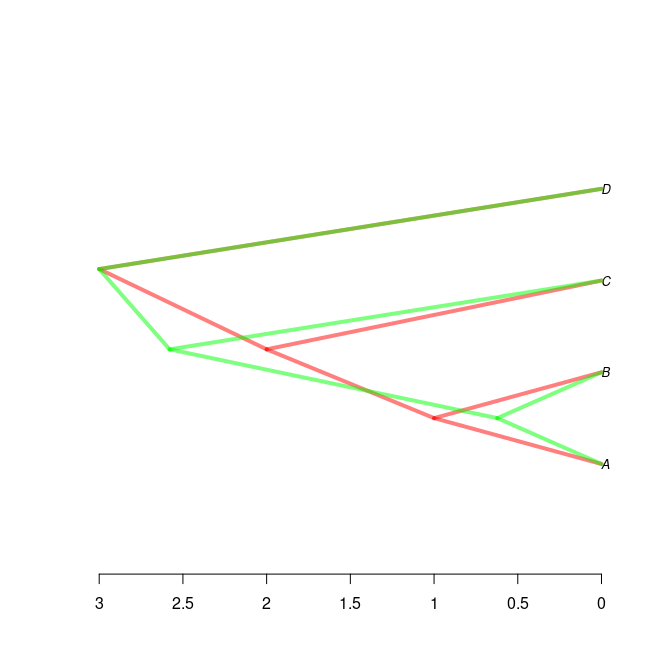# Create A Twin Tree

#### 2023-01-19

library(babette)
library(pirouette)
library(phangorn)
library(pryr)

First create a tree, then you can twin it. Currently there are two different way to do so. The first is generating the twin using a Birth-Death (bd) process. The second using a Yule process.

We pick this tree to twin:

phylogeny <- ape::read.tree(text = "(((A:1, B:1):1, C:2):1, D:3);")
ape::plot.phylo(phylogeny)The BD twinning parameters:

bd_twin <- create_twin_tree(
phylogeny = phylogeny,
twinning_params = create_twinning_params(
sim_twin_tree_fun = get_sim_bd_twin_tree_fun()
)
)

The Yule twinning parameters:

yule_twin <- create_twin_tree(
phylogeny = phylogeny,
twinning_params = create_twinning_params(
sim_twin_tree_fun = create_sim_yule_twin_tree_fun()
)
)

The copy twinning parameters:

copy_twin <- create_twin_tree(
phylogeny = phylogeny,
twinning_params = create_twinning_params(
sim_twin_tree_fun = create_copy_twtr_from_true_fun()
)
)

Show the true tree in red and bd twin tree in gray:

plot_densitree(
phylos = c(bd_twin, yule_twin, copy_twin),
col = c("red", "blue", "gray"),
width = 4,
consensus = phylogeny,
alpha = 0.5
)Show the true tree in red and bd twin tree in green:

plot_densitree(
phylos = c(phylogeny, yule_twin),
col = c("red", "green"),
width = 4,
consensus = phylogeny,
alpha = 0.5
)